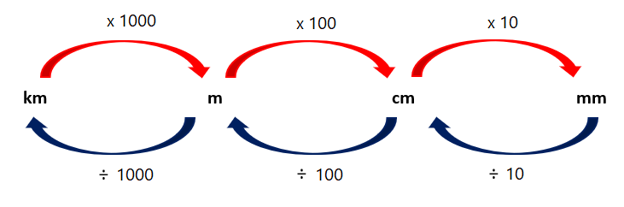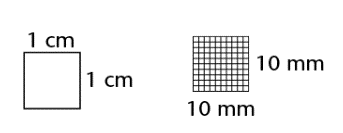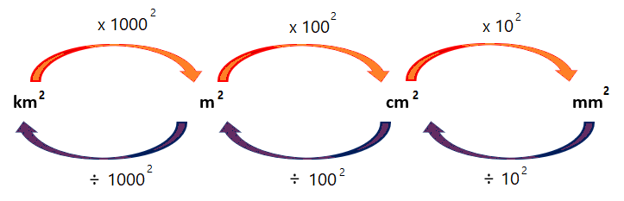Home Practice
For learners and parents For teachers and schools
Textbooks
Full catalogue
Pricing SupportLog in

We think you are located in United States. Is this correct?

# Units of length

## 16.2 Units of length

Lengths can be measured in millimetres (mm), centimetres (cm), metres (m) or kilometres (km). It is important to know how to convert between the different units of length.

Examples:

$1 \text{ cm} = 10 \text{ mm}$ $1 \text{ m} = 1\ 000 \text{ mm}$ $1 \text{ m} = 100 \text{ cm}$ $1 \text{ km} = 1\ 000 \text{ m}$

The diagram below shows how to convert between the different units of length.## Worked example 16.1: Converting between units of length

Convert $$\text{3,2} \text{ km}$$ to metres.

### Convert kilometres to metres.

$$\text{1} \text{ km} = \text{1 000} \text{ m}$$, so to convert kilometres to metres we must multiply by $$\text{1 000}$$.

$\text{3,2} \text{ km} = \text{3,2} \times \text{1 000} = \text{3 200} \text{ m}$

## Worked example 16.2: Converting between units of length

Convert $$\text{15,8} \text{ m}$$ to centimetres.

### Convert metres to centimetres.

$$\text{1} \text{ m} = \text{100} \text{ cm}$$, so to convert metres to centimetres we must multiply by $$\text{100}$$.

$\text{15,8} \text{ m} = \text{15,8} \times \text{100} = \text{1 580} \text{ cm}$

## Worked example 16.3: Converting between units of length

Convert $$\text{950} \text{ mm}$$ to metres.

### Convert millimetres to metres.

$$\text{1 000} \text{ mm} = \text{1} \text{ m}$$, so to convert millimetres to metres we must divide by $$\text{1 000}$$.

$\text{950} \text{ mm} = \text{950} \div \text{1 000} = \text{0,95} \text{ m}$

# Test yourself now

High marks in science are the key to your success and future plans. Test yourself and learn more on Siyavula Practice.

Exercise 16.1

Convert each length to centimetres.

1. $$\text{501} \text{ mm}$$
2. $$\text{2,4} \text{ km}$$
3. $$\text{17} \text{ m}$$
4. $$\text{78,3} \text{ mm}$$
5. $$\text{0,75} \text{ m}$$
1. $$\text{50,1} \text{ cm}$$
2. $$\text{240 000} \text{ cm}$$
3. $$\text{1 700} \text{ cm}$$
4. $$\text{7,83} \text{ cm}$$
5. $$\text{75} \text{ cm}$$

Convert each length to millimetres.

1. $$\text{2,15} \text{ m}$$
2. $$\text{14,8} \text{ cm}$$
3. $$\text{1,09} \text{ m}$$
4. $$\text{0,46} \text{ m}$$
5. $$\text{7,73} \text{ cm}$$
1. $$\text{2 150} \text{ mm}$$
2. $$\text{148} \text{ mm}$$
3. $$\text{1 090} \text{ mm}$$
4. $$\text{460} \text{ mm}$$
5. $$\text{77,3} \text{ mm}$$

Convert each length to metres.

1. $$\text{856} \text{ cm}$$
2. $$\text{2 071} \text{ mm}$$
3. $$\text{4,13} \text{ km}$$
4. $$\text{68,5} \text{ cm}$$
5. $$\text{759} \text{ mm}$$
1. $$\text{8,56} \text{ m}$$
2. $$\text{2,071} \text{ m}$$
3. $$\text{4 130} \text{ m}$$
4. $$\text{0,685} \text{ m}$$
5. $$\text{0,759} \text{ m}$$

Area is measured in square units. The diagram below shows a $$1 \text{ cm} \times 1 \text{ cm}$$ square which has an area of $$1 \text{ cm}^2$$. We know that $$1 \text{ cm} = 10 \text{ mm}$$. So the same square can be represented as a $$10 \text{ mm} \times 10 \text{ mm}$$ square, which has an area of $$100 \text{ mm}^2$$.

So, $$1 \text{ cm}^2 = 1 \times 10^2 \text{ mm}^2 = 100 \text{ mm}^2$$The diagram below shows how to convert between the different units of area.## Worked example 16.4: Converting between units of area

Convert $$255 \text{ mm}^2$$ to square centimetres.

### Convert square millimetres to square centimetres.

To convert from square millimetres to square centimetres, we divide the area by $$100$$.

$$\text{255} \text{ mm}^2 = \text{255} \div \text{100} = \text{2,55} \text{ cm}^2$$.

## Worked example 16.5: Converting between units of area

Convert $$\text{1,06} \text{ m}^2$$ to square centimetres.

### Convert square metres to square centimetres.

To convert from square metres to square centimetres, we multiply the area by $$100^2$$.

$$\text{1,06} \text{ m}^2 = \text{1,06} \times \text{100}^2 = \text{10 600} \text{ cm}^2$$.

# Test yourself now

High marks in science are the key to your success and future plans. Test yourself and learn more on Siyavula Practice.

Exercise 16.2

Convert each area to square centimetres.

1. $$\text{1,06} \text{ m}^2$$
2. $$\text{2 425} \text{ mm}^2$$
3. $$\text{0,035} \text{ m}^2$$
4. $$\text{341,5} \text{ mm}^2$$
5. $$\text{0,718} \text{ m}^2$$
1. $$\text{10 600} \text{ cm}^2$$
2. $$\text{24,25} \text{ cm}^2$$
3. $$\text{350} \text{ cm}^2$$
4. $$\text{3,415} \text{ cm}^2$$
5. $$\text{7 180} \text{ cm}^2$$

Convert each area to square millimetres.

1. $$\text{5,4} \text{ cm}^2$$
2. $$\text{0,002} \text{ m}^2$$
3. $$\text{0,95} \text{ cm}^2$$
4. $$\text{0,00014} \text{ m}^2\} 5. \(\text{12,8} \text{ cm}^2\} 1. \(\text{540} \text{ mm}^2$$
2. $$\text{2 000} \text{ mm}^2$$
3. $$\text{95} \text{ mm}^2$$
4. $$\text{1 400} \text{ mm}^2$$
5. $$\text{1 280} \text{ mm}^2$$

Convert each area to square metres.

1. $$\text{8 743} \text{ cm}^2$$
2. $$\text{45 000} \text{ mm}^2$$
3. $$\text{25 030} \text{ cm}^2$$
4. $$\text{6,01} \text{ km}^2$$
5. $$\text{650} \text{ cm}^2$$
1. $$\text{0,8743} \text{ m}^2$$
2. $$\text{0,045} \text{ m}^2$$
3. $$\text{2,503} \text{ m}^2$$
4. $$\text{6 010 000} \text{ m}^2$$
5. $$\text{0,065} \text{ m}^2$$